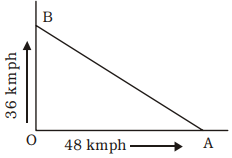Home » Aptitude » Speed, Time and Distance » Question

#### Speed, Time and Distance

1. Two cars start at the same time from one point and move along two roads at right angles to each other. Their speeds are 36 km/ hour and 48 km/hour respectively. After 15 seconds the distance between them will be
1. 400 m
2. 150 m
3. 300 m
4. 250 m
##### Correct Option: DLet O be the starting point. The car running at 36 kmph is moving along OB and that at 48 kmph moving along OA. Also let they reach at B and A respectively after 15 seconds.

 ∴   OA = 48 × 5 × 15 = 200 m 18

 ∴   and OB = 36 × 5 × 15 = 150 m 18

∴  Required distance = AB
= √(200)2 + (150)2
W (By Pythagoras theorem)
= √40000 + 22500
= √62500
= 250 m